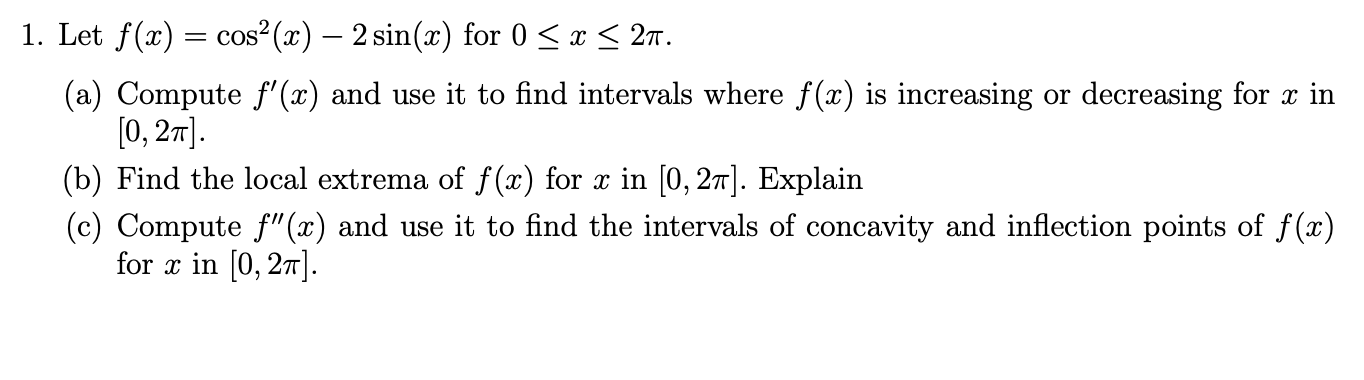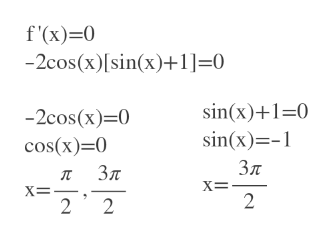# 1. Let f(x) cos2(x) - 2 sin(x) for 027x(a) Compute f'(x) and use it to find intervals where f(x) is increasing or decreasing for x in[0, 2m(b) Find the local extrema of f(x) for x in [0, 27]. Explain(c) Compute f"(x) and use it to find the intervals of concavity and inflection points of f(x)for in [0, 27

Question
174 views

Thank You!help_outlineImage Transcriptionclose1. Let f(x) cos2(x) - 2 sin(x) for 0 27 x (a) Compute f'(x) and use it to find intervals where f(x) is increasing or decreasing for x in [0, 2m (b) Find the local extrema of f(x) for x in [0, 27]. Explain (c) Compute f"(x) and use it to find the intervals of concavity and inflection points of f(x) for in [0, 27 fullscreen
check_circle

Step 1

First compute f'(x) using first derivative.

Step 2

Then find critical points using f'(x)=0.

Critical points are= pi/2 and 3pi/2help_outlineImage Transcriptionclosef'(x) 0 -2cos(x)[sin(x)+1]=0 sin(x)+1-0 sin(x)- -2cos(x) 0 cos(x) 0 Зл л Зл x=. 2 2 2 fullscreen
Step 3

Check sign of f\'(x) in each intervals.

f\'(pi/4) = negative

f\'(pi)=positive

f\'(7pi/4)= negative

So, in (0,pi/2) and (3pi/2 , 2pi) the function is decreasing. [because f\...

### Want to see the full answer?

See Solution

#### Want to see this answer and more?

Solutions are written by subject experts who are available 24/7. Questions are typically answered within 1 hour.*

See Solution
*Response times may vary by subject and question.
Tagged in
MathCalculus

### Other